nextnano.com  nextnano³  Download | Search | Copyright | Publications  * password protected nextnano³ software2D The CBR method (Transmission)

# nextnano3 - Tutorial

## Efficient method for the calculation of ballistic quantum transport - The CBR method (2D example)

Author: Stefan Birner

If you want to obtain the input files that are used within this tutorial, please check if you can find them in the installation directory.
If you cannot find them, please submit a Support Ticket.
```  -> 2D_CBR_MamaluySabathilJAP2003.in  (-> 2D_CBR_MamaluySabathilJAP2003_holes.in - ```same but for holes instead of electrons`)`

`==> `Download nextnano³ CBR test executable:``` 2D_CBR_Mamaluy_SabathilJAP2003.zip     ```(If you don't have login and passwort yet, please register here.)

## Efficient method for the calculation of ballistic quantum transport - The CBR method (2D example)

```  -> 2D_CBR_MamaluySabathilJAP2003.in  (-> 2D_CBR_MamaluySabathilJAP2003_holes.in - ```same but for holes instead of electrons`)`

In this tutorial we apply the Contact Block Reduction (CBR) method to a Aharonov-Bohm-type structure where there is a large barrier in the middle of the device.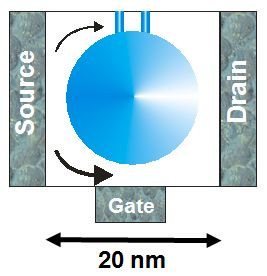schematic sketch of the device showing two possible paths of the electrons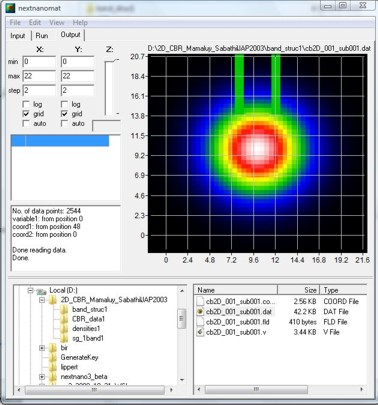nextnanomat screenshot (nextnanomat has been developed by Jörg Ehehalt)

This input file is based on the following paper:

`[SabathilJAP]`
Efficient method for the calculation of ballistic quantum transport
D. Mamaluy, M. Sabathil, P. Vogl
Journal of Applied Physics 93, 4628 (2003)

The device consists of three leads that are called 'source', 'gate' and 'drain' in this example.
In the middle of the device a potential barrier of two-dimensional Gaussian shape effectively expels the electrons from the center.
In the upper part of the device, a thin tunneling double barrier is present.
The device dimensions are 20 nm x 20 nm.
A detailed description of the device can be found in Section V. of``` [SabathilJAP]```.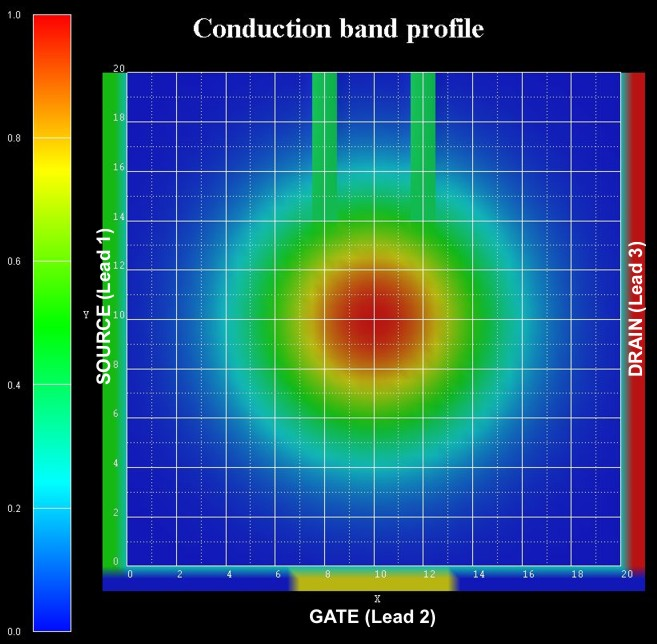The effective electron mass is assumed to be constant throughout the device and equal to 0.3 m0.
The device region consists of 41 x 41 = 1681 grid points, which is equivalent to a grid spacing of 0.5 nm.
This means that the device Hamiltonian is a matrix of size 1681 x 1681.

The tunneling barriers have a width of 1 nm each and are separated by 3 nm.
The maximum height of the Gaussian barrier is Ec,0 = 1 eV, the height of the double barriers is 0.4 eV.
The conduction band profile is given by Ec = Ec,0 exp[ `-` (x² + y²) / a² ] where x and y are with respect to the center of the device,
and a = 5 nm.
The conduction band profile is achieved by using an appropriate ternary material having a 2D Gaussian alloy profile.
The lower gate is 6 nm long, all other leads are 20 nm long.The following figure shows the calculated transmission coefficients of the various lead combinations T12, T23 and T13.
For the thick lines 18 % (303 of 1681) of all eigenvectors were used whereas for the thin lines only 7 % (118 of 1681) had to be calculated, i.e. one does not have to calculate all eigenvalues of the device Hamiltonian which grossly reduces CPU time.
A small percentage of eigenvalues suffices for T(E) in relevant energy range of interest.
The transmission coefficient can be found in this file:``` CBR_data1/transmission2D_cb_sg001_ind000_CBR.dat```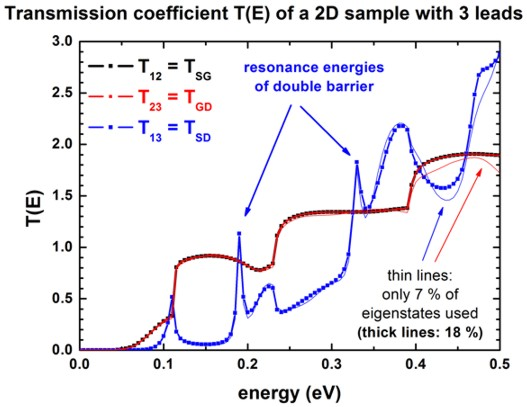The nextnano³ results differ slightly from the``` [SabathilJAP] ```paper.
Reason: The potential energy profile in the device and in the leads is not exactly identical, as well as the dimensions of the barriers.
Therefore the eigenenergies and wave functions in the device, and in the leads differ slightly which explains the small deviations.

` ==> `The eigenstates # 16 is a resonance state of the lower transmission path.```      1st resonance: # 16: 0.123 eV      ```Its square of the wave function is shown below.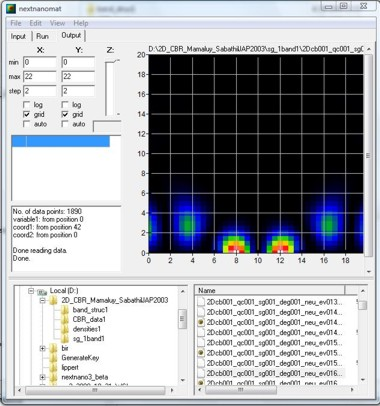nextnanomat screenshot (nextnanomat has been developed by Jörg Ehehalt)

` ==> `The eigenstates # 26 and # 29 are resonance states of the double barrier```.      1st resonance: # 26: 0.182 eV (delocalized)                     # 29: 0.196 eV (more localized)      2nd resonance: # 55: 0.322 eV (delocalized)                     # 57: 0.333 eV (more localized)                     # 60: 0.345 eV (delocalized)                     # 63: 0.359 eV (more localized)```

The following figure shows the conduction band profile together with the square of the wave function of the 26th eigenstate.
One can clearly see that it is a resonance state of the double barrier and corresponds to the second peak in the blue transmission curve T13 from source to drain around 180 meV.
``` ```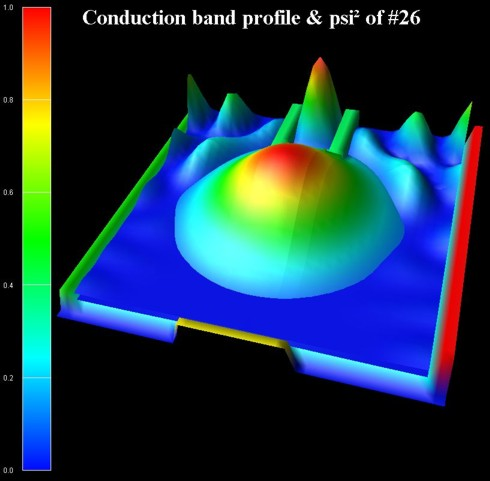The following two figures show the lead modes of the gate, and the source (which is identical to the drain).
In the transmission curve T12(E) = T23(E), the transmission shows a step-like behavior which is related to the energies of lead no. 2 ('gate').

The lead modes (eigenvalues, psi, psi², band edge profile, ...) can be found in these files:```   CBR_data1/modes_lead00*_sg001_*.dat```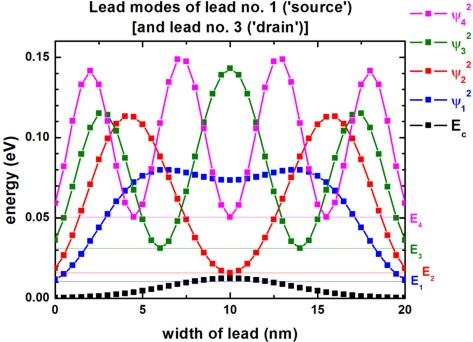## Technical details

### Definition of contacts

For each contact (lead), a quantum cluster ("lead quantum cluster") has to be defined because in each lead,
a one-dimensional Schrödinger equation has to be solved which gives us the lead modes (i.e. energies and eigenvectors of the leads).
In addition, a quantum cluster is required for the device itself ("main quantum cluster").

``` !---------------------------------------------------!  \$quantum-regions                                    !   region-number   = 1                                ! 'device'   base-geometry   = rectangle                        !   region-priority = 2                                !   x-coordinates   = 0d0 20d0                         ! [nm]  width of 'device' = 20 nm   y-coordinates   = 0d0 20d0                         ! [nm]  width of 'device' = 20 nm   region-number   = 2                                ! 'source'   base-geometry   = rectangle                        !   region-priority = 1                                !   x-coordinates   = -1d0 -0.5d0                      ! [nm]  ```(including 2 grid points along this direction)```   y-coordinates   =  0d0 20d0                        ! [nm]  length of 'lead 1' = 20 nm   region-number   = 3                                ! 'gate'   base-geometry   = rectangle                        !   region-priority = 1                                !   x-coordinates   =  7d0 13d0                        ! [nm]  length of 'lead 2' =  6 nm   y-coordinates   = -1d0 -0.5d0                      ! [nm]  ```(including 2 grid points along this direction)```   region-number   = 4                                ! 'drain'   base-geometry   = rectangle                        !   region-priority = 1                                !   x-coordinates   = 20.5d0 21d0                      ! [nm]  ```(including 2 grid points along this direction)```   y-coordinates   = 0d0    20d0                      ! [nm]  length of 'lead 3' = 20 nm  \$end_quantum-regions                                !  !---------------------------------------------------!  ```

For each quantum cluster, the number of eigenstates to be calculated and its boundary conditions have to be specified.

``` !-------------------------------------------------!  \$quantum-model-electrons                          !   model-number                   = 1               !   model-name                     = effective-mass  ! ```single band effective-mass Schrödinger equation```   cluster-numbers                = 1   conduction-band-numbers        = 1               ! ```Gamma band ```  !number-of-eigenvalues-per-band = 118             ! ```corresponds to   7.0 % of 1681```   number-of-eigenvalues-per-band = 303             ! ```corresponds to  18.0 % of 1681```  !number-of-eigenvalues-per-band = 1681            ! ```corresponds to 100.0 % of 1681```   quantization-along-axes        = 1 1 0           ! ```(x,y)-plane```   boundary-condition-100         = Neumann         ! ```Neumann along propagation direction```   boundary-condition-010         = Neumann         ! ```Neumann along propagation direction

For the main quantum cluster it holds:``` ```For each grid point in the main quantum cluster it is checked if it is at the boundary and if it is in contact to a lead.
If it is at the boundary, and if it is       in contact to a lead, a Neumann boundary condition is set.
If it is at the boundary, and if it is not in contact to a lead, a Dirichlet          boundary condition is set.

``` !-------------------------------------------------!  ! lead 1 = source: number of modes per lead = 41 =  ```maximum number of relevant quantum grid points in lead 1```  !-------------------------------------------------!   model-number                   = 2               !   ...   cluster-numbers                = 2               !   number-of-eigenvalues-per-band = 41              ! ```calculate 41 lead modes```   boundary-condition-010         = Dirichlet       ! ```Dirichlet perpendicular to propagation direction```  !-------------------------------------------------!  ! lead 2 = gate:   number of modes per lead = 13 = ```maximum number of relevant quantum grid points in lead 2```  !-------------------------------------------------!   model-number                   = 3               !   ...   cluster-numbers                = 2               !   number-of-eigenvalues-per-band = 13              ! ```calculate 13 lead modes```   boundary-condition-100         = Dirichlet       ! ```Dirichlet perpendicular to propagation direction```  !-------------------------------------------------!  ! lead 3 = drain:  number of modes per lead = 41 = ```maximum number of relevant quantum grid points in lead 3```  !-------------------------------------------------!   model-number                   = 4               !   ...   cluster-numbers                = 2               !   number-of-eigenvalues-per-band = 41              ! ```calculate 41 lead modes```   boundary-condition-010         = Dirichlet       ! ```Dirichlet perpendicular to propagation direction```  \$end_quantum-model-electrons                      !  !-------------------------------------------------!  ```

``` !-------------------------------------------------!  \$CBR-current                                      !                                                    !   destination-directory = CBR_data1/               ! ```directory for output and data files```   calculate-CBR         = yes                      ! ```flag:``` "yes"/"no"                                                    !   main-qr-num           = 1                        ! ```number of main quantum cluster for which transport is calculated:``` 'device'   num-leads             = 3                        ! ```total number of leads attached to main region```   lead-qr-numbers       = 2  3  4                  ! ```quantum cluster numbers corresponding to each lead```   propagation-direction = 1  2  1 ! '1'=x, '2'=y   ! ```direction of propagation (`1`,`2`,`3`) for  each lead```   num-modes-per-lead    = 41 13 41                 ! ```number of modes per lead used for CBR calculation
```                                                   ! ```must be` <= `number of eigenvalues specified in corresponding quantum model ```  !num-eigenvectors-used = 118                      ! ```corresponds to   7.0 % of 1681```   num-eigenvectors-used = 303                      ! ```corresponds to 18.0 % of 1681```  !num-eigenvectors-used = 1681 ! 1681=41*41=N_x*N_y! ```number of eigenvectors in main quantum cluster used for CBR calculation```   E-min                 = 0.0d0                    ! [eV] ```lower boundary for transmission energy interval```   E-max                 = 0.5d0                    ! [eV] ```upper boundary for transmission energy interval```   num-energy-steps      = 100                      ! ```number of energy steps in T(E)```                                                    !  \$end_CBR-current                                  !  !-------------------------------------------------!  ```

For each energy E (`num-energy-steps = 100`) where the transmission coefficient T(E) has to be calculated,
a matrix of size 95 x 95 has to be inverted.
The size of 95 is determined by the sum of the number of grid points in each lead that are in contact to the device.

• Lead 1 (Source): 41 grid points
• Lead 2 (Gate):    13 grid points
• Lead 3 (Drain):    41 grid points

`==> `in total: 95 grid points

`==> `The total CPU time for calculation of the transmission T(E) in this example was about 30 seconds for 303 eigenstates.

For further information, please study this section:``` \$CBR-current```